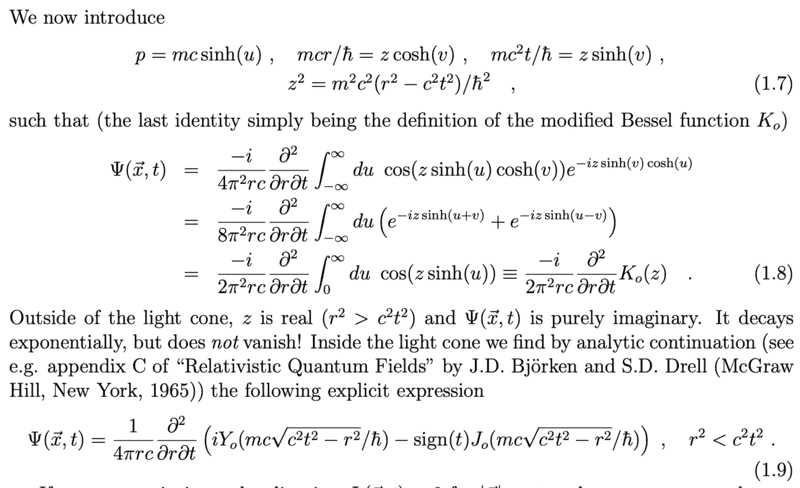# Help with simplifying an integral

TroyElliott
TL;DR Summary
Stuck on some algebra within an integral.
I am not seeing how the v goes away in the third equal sign of equation (1.8). It seems to be that it must be cos(z*sinh(u+v)), not cos(z*sinh(u)).
In the defined equations (1.7), the variable "v" can become imaginary, so a simple change of variables would change the integration sign by adding an imaginary term - not what occurs in (1.8). Any idea on how to get the third line of (1.8)?

Thanks for any insight!Gold Member
In order to get the third line in Eq. (1.8) change variables ##u+v\mapsto u## and ##u-v\mapsto u##.
Since ##v## is constant with respect to the integration varible ##du = d(u\pm v)##, and also don't forget that integral of an even function ##f(x)=f(-x)## leads to ##\int_{-a}^a f(x)dx= 2\int_0^a f(x)dx##.

•anuttarasammyak
TroyElliott
In order to get the third line in Eq. (1.8) change variables ##u+v\mapsto u## and ##u-v\mapsto u##.
Since ##v## is constant with respect to the integration varible ##du = d(u\pm v)##, and also don't forget that integral of an even function ##f(x)=f(-x)## leads to ##\int_{-a}^a f(x)dx= 2\int_0^a f(x)dx##.

But, in this case, doesn’t it matter than ##v## can potentially become an imaginary number, and the limits of the integral would no longer be just infinity? We would get an added imaginary term to the limit, e.g. ##\infty + 2\pi i##.

More explicitly, ##v= arcsinh(\frac{mc^{2}t}{hz}),## where ##z## is given in Eq.(1.7). We see that inside the light cone, ##z## becomes imaginary. So ##v## would also become imaginary, in this case. When performing the change of variables, the integration bound would now pick up an additional imaginary piece. This is what I am confused about - how can it still just be ##\infty##?

Last edited:
Gold Member
u and v are both real and v is constant as for integration of u.
$$\int_{-\infty}^{+\infty} du = \int_{-\infty}^{+\infty} d(u+v)$$
or so.

TroyElliott
In order to get the third line in Eq. (1.8) change variables ##u+v\mapsto u## and ##u-v\mapsto u##.
Since ##v## is constant with respect to the integration varible ##du = d(u\pm v)##, and also don't forget that integral of an even function ##f(x)=f(-x)## leads to ##\int_{-a}^a f(x)dx= 2\int_0^a f(x)dx##.
u and v are both real and v is constant as for integration of u.
$$\int_{-\infty}^{+\infty} du = \int_{-\infty}^{+\infty} d(u+v)$$
or so.
But we see in Eq.(1.7), that inside the light cone, ##v## can become imaginary.

Gold Member
It seems that (1.8) is for real z with u and v real, and (1.9) is for imaginary z.

•TroyElliott
Gold Member
But we see in Eq.(1.7), that inside the light cone, ##v## can become imaginary.
Such a calculation is done in a course in complex analysis; I don't recall the details, sorry.

•TroyElliott